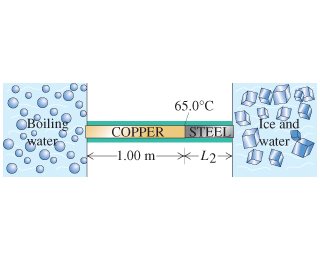# Problem: A long rod, insulated to prevent heat loss along its sides, is in perfect thermal contact with boiling water (at atmospheric pressure) at one end and with an ice-water mixture at the other. The rod consists of a 1.00-m-long section of copper (one end in steam) joined end-to-end to a length of steel (one end in ice). Both sections of the rod have cross-section areas of 4.00 cm2. The temperature of the copper-steel junction is 65.0 °C after a steady state has been set up. Assume that the thermal conductivities of copper and steel are given by kcopper = 385 W/(m⋅K) and ksteel = 50.2 W/(m⋅K).How much heat per second flows from the steambath to the ice-water mixture?

###### FREE Expert Solution

Amount of heat:

$\overline{){\mathbf{Q}}{\mathbf{=}}\frac{\mathbf{k}\mathbf{A}\mathbf{∆}\mathbf{T}\mathbf{t}}{\mathbf{L}}}$

The rate of heat flow per second is:

H = Q/t, from the above equation.###### Problem Details

A long rod, insulated to prevent heat loss along its sides, is in perfect thermal contact with boiling water (at atmospheric pressure) at one end and with an ice-water mixture at the other. The rod consists of a 1.00-m-long section of copper (one end in steam) joined end-to-end to a length of steel (one end in ice). Both sections of the rod have cross-section areas of 4.00 cm2. The temperature of the copper-steel junction is 65.0 °C after a steady state has been set up. Assume that the thermal conductivities of copper and steel are given by kcopper = 385 W/(m⋅K) and ksteel = 50.2 W/(m⋅K).How much heat per second flows from the steambath to the ice-water mixture?